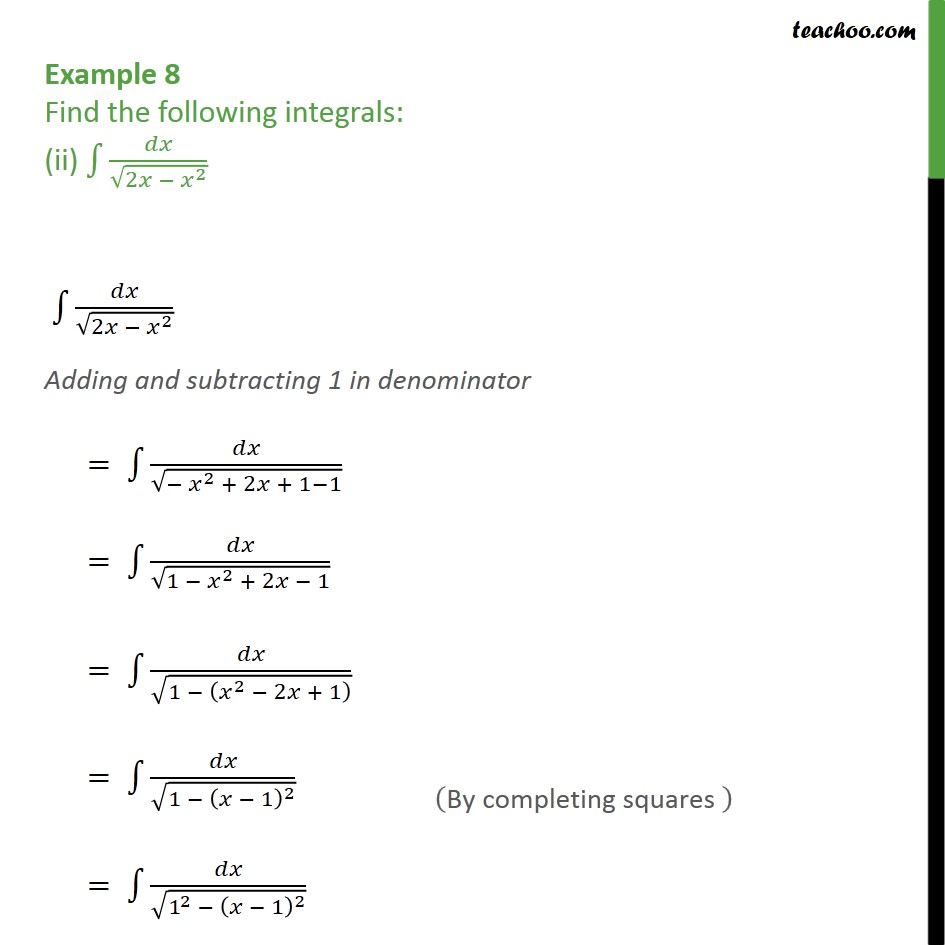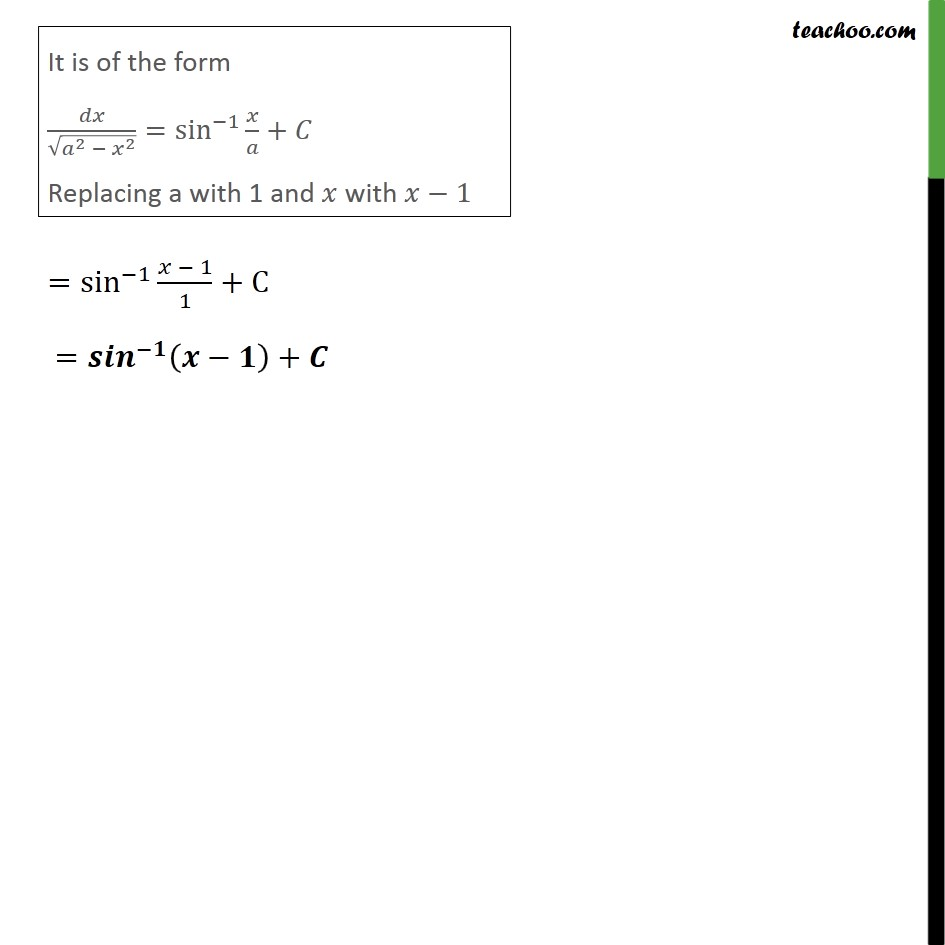Integration by specific formulaes - Formula 5

Chapter 7 Class 12 Integrals
Concept wiseLearn in your speed, with individual attention - Teachoo Maths 1-on-1 Class

### Transcript

Example 8 Find the following integrals: (ii) 1 / (2 ^2 ) 1 / (2 ^2 ) Adding and subtracting 1 in denominator = 1 / ( ^2 + 2 + 1 1) = 1 / (1 ^2 + 2 1) = 1 / (1 ( ^2 2 + 1) ) = 1 / (1 ( 1) ^2 ) = 1 / (1^2 ( 1) ^2 )